Ionization Energy and Electron Affinity

The First Ionization Energy

The energy needed to remove one or more electrons from a neutral atom to form a positively charged ion is a physical property that influences the chemical behavior of the atom. By definition, the first ionization energy of an element is the energy needed to remove the outermost, or highest energy, electron from a neutral atom in the gas phase.

The process by which the first ionization energy of hydrogen is measured would be represented by the following equation.

 H(g)H+(g) + e-Ho = -1312.0 kJ/mol

 Practice Problem 3:Use the Bohr model to calculate the wavelength and energy of the photon that would have to be absorbed to ionize a neutral hydrogen atom in the gas phase. Click here to check your answer to Practice Problem 3 Click here to see a solution to Practice Problem 3

The magnitude of the first ionization energy of hydrogen can be brought into perspective by comparing it with the energy given off in a chemical reaction. When we burn natural gas, about 800 kJ of energy is released per mole of methane consumed.

 CH4(g) + 2 O2(g)CO2(g) + 2 H2O(g)Ho = -802.4 kJ/mol

The thermite reaction, which is used to weld iron rails, gives off about 850 kJ of energy per mole of iron oxide consumed.

 Fe2O3(s) + 2 Al(s)Al2O3(s) + 2 Fe(l)Ho = -851.5 kJ/mol

The first ionization energy of hydrogen is half again as large as the energy given off in either of these reactions.Patterns in the First Ionization Energies

The first ionization energy for helium is slightly less than twice the ionization energy for hydrogen because each electron in helium feels the attractive force of two protons, instead of one.

 He(g)He+(g) + e-Ho = 2372.3 kJ/mol

It takes far less energy, however, to remove an electron from a lithium atom, which has three protons in its nucleus.

 Li(g)Li+(g) + e-Ho = 572.3 kJ/mol

This can be explained by noting that the outermost, or highest energy, electron on a lithium atom is in the 2s orbital. Because the electron in a 2s orbital is already at a higher energy than the electrons in a 1s orbital, it takes less energy to remove this electron from the atom.

The first ionization energies for the main group elements are given in the two figures below.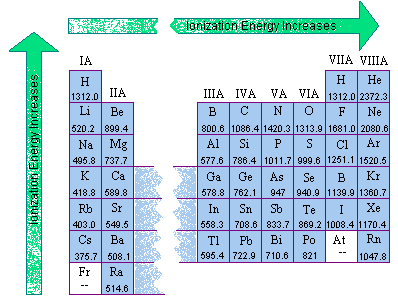Two trends are apparent from these data.

• In general, the first ionization energy increases as we go from left to right across a row of the periodic table.
• The first ionization energy decreases as we go down a column of the periodic table.

The first trend isn't surprising. We might expect the first ionization energy to become larger as we go across a row of the periodic table because the force of attraction between the nucleus and an electron becomes larger as the number of protons in the nucleus of the atom becomes larger.

The second trend results from the fact that the principal quantum number of the orbital holding the outermost electron becomes larger as we go down a column of the periodic table. Although the number of protons in the nucleus also becomes larger, the electrons in smaller shells and subshells tend to screen the outermost electron from some of the force of attraction of the nucleus. Furthermore, the electron being removed when the first ionization energy is measured spends less of its time near the nucleus of the atom, and it therefore takes less energy to remove this electron from the atom.Exceptions to the General Pattern of First Ionization Energies

The figure below shows the first ionization energies for elements in the second row of the periodic table. Although there is a general trend toward an increase in the first ionization energy as we go from left to right across this row, there are two minor inversions in this pattern. The first ionization energy of boron is smaller than beryllium, and the first ionization energy of oxygen is smaller than nitrogen.These observations can be explained by looking at the electron configurations of these elements. The electron removed when a beryllium atom is ionized comes from the 2s orbital, but a 2p electron is removed when boron is ionized.

Be: [He] 2s2

B: [He] 2s2 2p1

The electrons removed when nitrogen and oxygen are ionized also come from 2p orbitals.

N: [He] 2s2 2p3

O: [He] 2s2 2p4

But there is an important difference in the way electrons are distributed in these atoms. Hund's rules predict that the three electrons in the 2p orbitals of a nitrogen atom all have the same spin, but electrons are paired in one of the 2p orbitals on an oxygen atom.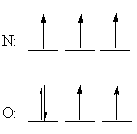Hund's rules can be understood by assuming that electrons try to stay as far apart as possible to minimize the force of repulsion between these particles. The three electrons in the 2p orbitals on nitrogen therefore enter different orbitals with their spins aligned in the same direction. In oxygen, two electrons must occupy one of the 2p orbitals. The force of repulsion between these electrons is minimized to some extent by pairing the electrons. There is still some residual repulsion between these electrons, however, which makes it slightly easier to remove an electron from a neutral oxygen atom than we would expect from the number of protons in the nucleus of the atom.

 Practice Problem 4:Predict which element in each of the following pairs has the larger first ionization energy. (a) Na or Mg (b) Mg or Al (b) Mg or Al (c) F or Cl Click here to check your answer to Practice Problem 4Second, Third, Fourth, and Higher Ionization Energies

By now you know that sodium forms Na+ ions, magnesium forms Mg2+ ions, and aluminum forms Al3+ ions. But have you ever wondered why sodium doesn't form Na2+ ions, or even Na3+ ions? The answer can be obtained from data for the second, third, and higher ionization energies of the element.

The first ionization energy of sodium, for example, is the energy it takes to remove one electron from a neutral atom.

Na(g) + energyNa+(g) + e-

The second ionization energy is the energy it takes to remove another electron to form an Na2+ ion in the gas phase.

Na+(g) + energyNa2+(g) + e-

The third ionization energy can be represented by the following equation.

Na2+(g) + energyNa3+(g) + e-

The energy required to form a Na3+ ion in the gas phase is the sum of the first, second, and third ionization energies of the element.

First, Second, Third, and Fourth Ionization Energies
of Sodium, Magnesium, and Aluminum (kJ/mol)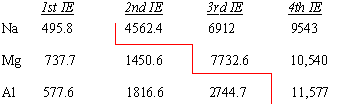It doesn't take much energy to remove one electron from a sodium atom to form an Na+ ion with a filled-shell electron configuration. Once this is done, however, it takes almost 10 times as much energy to break into this filled-shell configuration to remove a second electron. Because it takes more energy to remove the second electron than is given off in any chemical reaction, sodium can react with other elements to form compounds that contain Na+ ions but not Na2+ or Na3+ ions.

A similar pattern is observed when the ionization energies of magnesium are analyzed. The first ionization energy of magnesium is larger than sodium because magnesium has one more proton in its nucleus to hold on to the electrons in the 3s orbital.

Mg: [Ne] 3s2

The second ionization energy of Mg is larger than the first because it always takes more energy to remove an electron from a positively charged ion than from a neutral atom. The third ionization energy of magnesium is enormous, however, because the Mg2+ ion has a filled-shell electron configuration.

The same pattern can be seen in the ionization energies of aluminum. The first ionization energy of aluminum is smaller than magnesium. The second ionization energy of aluminum is larger than the first, and the third ionization energy is even larger. Although it takes a considerable amount of energy to remove three electrons from an aluminum atom to form an Al3+ ion, the energy needed to break into the filled-shell configuration of the Al3+ ion is astronomical. Thus, it would be a mistake to look for an Al4+ ion as the product of a chemical reaction.

 Practice Problem 5:Predict the group in the periodic table in which an element with the following ionization energies would most likely be found. 1st IE = 786 kJ/mol 2nd IE = 1577 3rd IE = 3232 4th IE = 4355 5th IE = 16,091 6th IE = 19,784 Click here to check your answer to Practice Problem 5

 Practice Problem 6:Use the trends in the ionization energies of the elements to explain the following observations. (a) Elements on the left side of the periodic table are more likely than those on the right to form positive ions. (b) The maximum positive charge on an ion is equal to the group number of the element Click here to check your answer to Practice Problem 6Electron Affinity

Ionization energies measure the tendency of a neutral atom to resist the loss of electrons. It takes a considerable amount of energy, for example, to remove an electron from a neutral fluorine atom to form a positively charged ion.

 F(g)F+(g) + e-Ho = 1681.0 kJ/mol

The electron affinity of an element is the energy given off when a neutral atom in the gas phase gains an extra electron to form a negatively charged ion. A fluorine atom in the gas phase, for example, gives off energy when it gains an electron to form a fluoride ion.

 F(g) + e-F-(g)Ho = -328.0 kJ/mol

Electron affinities are more difficult to measure than ionization energies and are usually known to fewer significant figures. The electron affinities of the main group elements are shown in the figure below.Several patterns can be found in these data.

• Electron affinities generally become smaller as we go down a column of the periodic table for two reasons. First, the electron being added to the atom is placed in larger orbitals, where it spends less time near the nucleus of the atom. Second, the number of electrons on an atom increases as we go down a column, so the force of repulsion between the electron being added and the electrons already present on a neutral atom becomes larger.
• Electron affinity data are complicated by the fact that the repulsion between the electron being added to the atom and the electrons already present on the atom depends on the volume of the atom. Among the nonmetals in Groups VIA and VIIA, this force of repulsion is largest for the very smallest atoms in these columns: oxygen and fluorine. As a result, these elements have a smaller electron affinity than the elements below them in these columns as shown in the figure below. From that point on, however, the electron affinities decrease as we continue down these columns.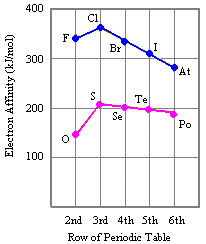At first glance, there appears to be no pattern in electron affinity across a row of the periodic table, as shown in the figure below.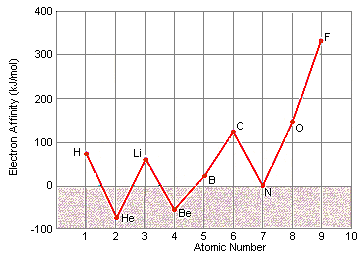When these data are listed along with the electron configurations of these elements, however, they make sense. These data can be explained by noting that electron affinities are much smaller than ionization energies. As a result, elements such as helium, beryllium, nitrogen, and neon, which have unusually stable electron configurations, have such small affinities for extra electrons that no energy is given off when a neutral atom of these elements picks up an electron. These configurations are so stable that it actually takes energy to force one of these elements to pick up an extra electron to form a negative ion.

Electron Affinities and Electron Configurations for the First 10 Elements in the Periodic Table

 Element Electron Affinity (kJ/mol) Electron Configuration H 72.8 1s1 He <0 1s2 Li 59.8 [He] 2s1 Be <0 [He] 2s2 B 27 [He] 2s2 2p1 C 122.3 [He] 2s2 2p2 N <0 [He] 2s2 2p3 O 141.1 [He] 2s2 2p4 F 328.0 [He] 2s2 2p5 Ne <0 [He] 2s2 2p6Consequences of the Relative Size of Ionization Energies and Electron Affinities

Students often believe that sodium reacts with chlorine to form Na+ and Cl- ions because chlorine atoms "like" electrons more than sodium atoms do. There is no doubt that sodium reacts vigorously with chlorine to form NaCl.

2 Na(s) + Cl2(g)2 NaCl(s)

Furthermore, the ease with which solutions of NaCl in water conduct electricity is evidence for the fact that the product of this reaction is a salt, which contains Na+ and Cl- ions.

 NaCl(s) H2ONa+(aq) + Cl-(aq)

The only question is whether it is legitimate to assume that this reaction occurs because chlorine atoms "like" electrons more than sodium atoms.

The first ionization energy for sodium is one and one-half times larger than the electron affinity for chlorine.

Na: 1st IE = 495.8 kJ/mol

Cl: EA = 328.8 kJ/mol

Thus, it takes more energy to remove an electron from a neutral sodium atom than is given off when the electron is picked up by a neutral chlorine atom. We will obviously have to find another explanation for why sodium reacts with chlorine to form NaCl. Before we can do this, however, we need to know more about the chemistry of ionic compounds.